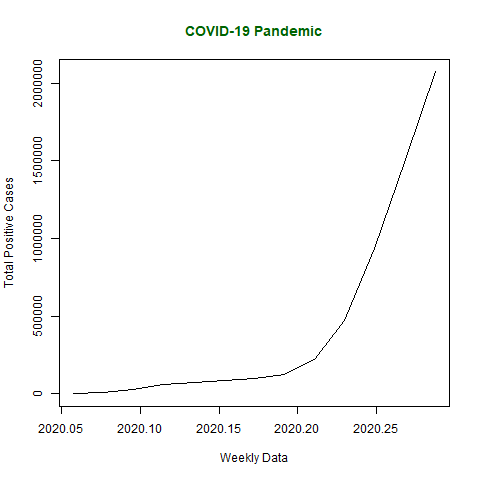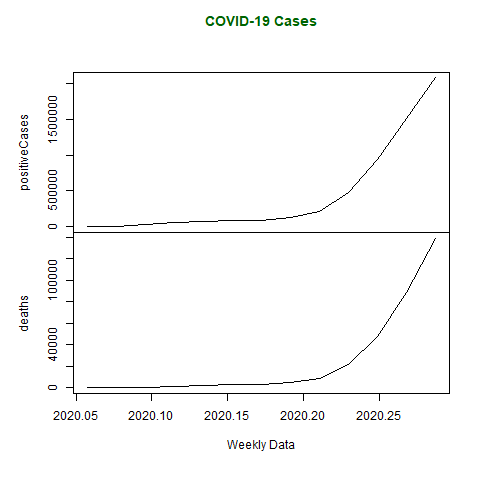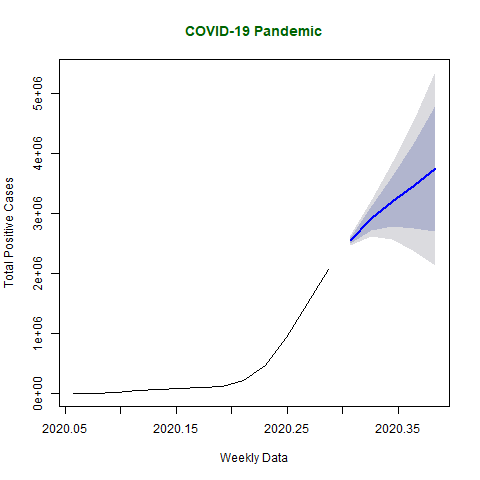# Time Series Analysis in R

• Difficulty Level : Easy
• Last Updated : 16 Dec, 2021

Time Series in R is used to see how an object behaves over a period of time. In R, it can be easily done by ts() function with some parameters. Time series takes the data vector and each data is connected with timestamp value as given by the user. This function is mostly used to learn and forecast the behavior of an asset in business for a period of time. For example, sales analysis of a company, inventory analysis, price analysis of a particular stock or market, population analysis, etc.

Syntax:  objectName <- ts(data, start, end, frequency)

where,

• data represents the data vector
• start represents the first observation in time series
• end represents the last observation in time series
• frequency represents number of observations per unit time. For example, frequency=1 for monthly data.

Note: To know about more optional parameters, use the following command in R console:

`help("ts")`

Example: Let’s take the example of COVID-19 pandemic situation. Taking a total number of positive cases of COVID-19 cases weekly from 22 January 2020 to 15 April 2020 of the world in data vector.

## R

 `# Weekly data of COVID-19 positive cases from` `# 22 January, 2020 to 15 April, 2020` `x <- ``c``(580, 7813, 28266, 59287, 75700,` `    ``87820, 95314, 126214, 218843, 471497,` `    ``936851, 1508725, 2072113)`   `# library required for decimal_date() function` `library``(lubridate)`   `# output to be created as png file` `png``(file =``"timeSeries.png"``)`   `# creating time series object` `# from date 22 January, 2020` `mts <- ``ts``(x, start = ``decimal_date``(``ymd``(``"2020-01-22"``)),` `                            ``frequency = 365.25 / 7)`   `# plotting the graph` `plot``(mts, xlab =``"Weekly Data"``,` `        ``ylab =``"Total Positive Cases"``,` `        ``main =``"COVID-19 Pandemic"``,` `        ``col.main =``"darkgreen"``)`   `# saving the file` `dev.off``()`

Output:## Multivariate Time Series

Multivariate Time Series is creating multiple time series in a single chart.

Example: Taking data of total positive cases and total deaths from COVID-19 weekly from 22 January 2020 to 15 April 2020 in data vector.

## R

 `# Weekly data of COVID-19 positive cases and` `# weekly deaths from 22 January, 2020 to` `# 15 April, 2020` `positiveCases <- ``c``(580, 7813, 28266, 59287,` `                ``75700, 87820, 95314, 126214,` `                ``218843, 471497, 936851,` `                ``1508725, 2072113)`   `deaths <- ``c``(17, 270, 565, 1261, 2126, 2800,` `            ``3285, 4628, 8951, 21283, 47210,` `            ``88480, 138475)`   `# library required for decimal_date() function` `library``(lubridate)`   `# output to be created as png file` `png``(file =``"multivariateTimeSeries.png"``)`   `# creating multivariate time series object` `# from date 22 January, 2020` `mts <- ``ts``(``cbind``(positiveCases, deaths),` `start = ``decimal_date``(``ymd``(``"2020-01-22"``)),` `                    ``frequency = 365.25 / 7)`   `# plotting the graph` `plot``(mts, xlab =``"Weekly Data"``,` `    ``main =``"COVID-19 Cases"``,` `    ``col.main =``"darkgreen"``)`   `# saving the file` `dev.off``()`

Output:## Forecasting

Forecasting can be done on time series using some models present in R. In this example, Arima automated model is used. To know about more parameters of arima() function, use the below command.

`help("arima")`

In the below code, forecasting is done using forecast library and so, installation of forecast library is necessary.

## R

 `# Weekly data of COVID-19 cases from` `# 22 January, 2020 to 15 April, 2020` `x <- ``c``(580, 7813, 28266, 59287, 75700,` `    ``87820, 95314, 126214, 218843,` `    ``471497, 936851, 1508725, 2072113)`   `# library required for decimal_date() function` `library``(lubridate)`   `# library required for forecasting` `library``(forecast)`   `# output to be created as png file` `png``(file =``"forecastTimeSeries.png"``)`   `# creating time series object` `# from date 22 January, 2020` `mts <- ``ts``(x, start = ``decimal_date``(``ymd``(``"2020-01-22"``)),` `                            ``frequency = 365.25 / 7)`   `# forecasting model using arima model` `fit <- ``auto.arima``(mts)`   `# Next 5 forecasted values` `forecast``(fit, 5)`   `# plotting the graph with next` `# 5 weekly forecasted values` `plot``(``forecast``(fit, 5), xlab =``"Weekly Data"``,` `ylab =``"Total Positive Cases"``,` `main =``"COVID-19 Pandemic"``, col.main =``"darkgreen"``)`   `# saving the file` `dev.off``()`

Output :
After executing the above code, the following forecasted results are produced.

```         Point Forecast   Lo 80   Hi 80   Lo 95   Hi 95
2020.307        2547989 2491957 2604020 2462296 2633682
2020.326        2915130 2721277 3108983 2618657 3211603
2020.345        3202354 2783402 3621307 2561622 3843087
2020.364        3462692 2748533 4176851 2370480 4554904
2020.383        3745054 2692884 4797225 2135898 5354210```

Below graph plots estimated forecasted values of COVID-19 if it continues to be widespread for the next 5 weeks.My Personal Notes arrow_drop_up
Recommended Articles
Page :